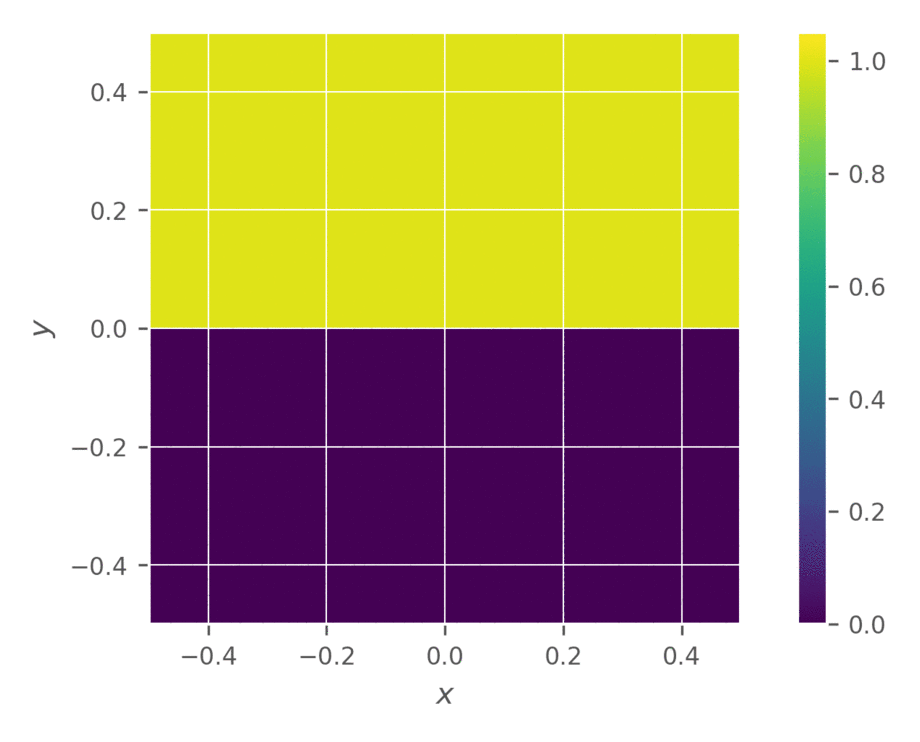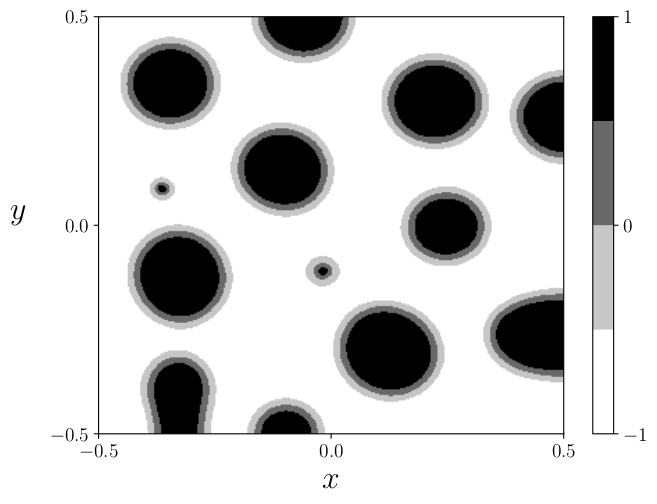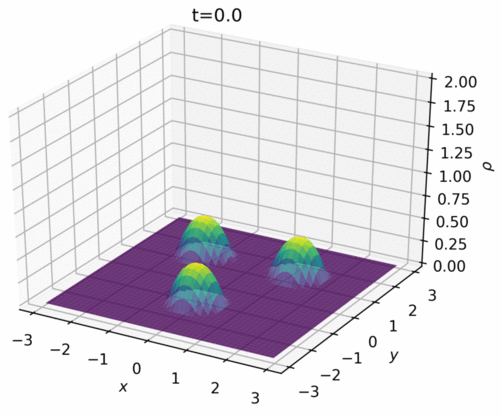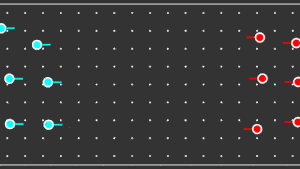## Publications

#### Projective and telescopic projective integration for non-linear kinetic mixtures

Rafael Bailo
Thomas Rey

Journal of Computational Physics, 458, 2022.

@Article{BR2022
title={Projective and telescopic projective integration for non-linear kinetic mixtures},
author={Bailo, Rafael and Rey, Thomas},
journal={J Comput Phys},
year={2022},
doi={10.1016/j.jcp.2022.111082},
volume={458},
archivePrefix={arXiv},
arxivId={2106.08811},
eprint={2106.08811},
}

We propose fully explicit projective integration and telescopic projective integration schemes for the multispecies Boltzmann and Bhatnagar-Gross-Krook (BGK) equations. The methods employ a sequence of small forward-Euler steps, intercalated with large extrapolation steps. The telescopic approach repeats said extrapolations as the basis for an even larger step. This hierarchy renders the computational complexity of the method essentially independent of the stiffness of the problem, which permits the eﬃcient solution of equations in the hyperbolic scaling with very small Knudsen numbers. We validate the schemes on a range of scenarios, demonstrating its prowess in dealing with extreme mass ratios, ﬂuid instabilities, and other complex phenomena.#### Bound-preserving finite-volume schemes for systems of continuity equations with saturation

arXiv: 2110.08186, 2021.

@Article{BCH2021
title={Bound-preserving finite-volume schemes for systems of continuity equations with saturation},
author={Bailo, Rafael and Carrillo, José Antonio and Hu, Jingwei},
journal={arXiv: 2110.08186},
year={2021},
doi={10.48550/arXiv.2110.08186},
archivePrefix={arXiv},
arxivId={2110.08186},
eprint={2110.08186},
}

We propose finite-volume schemes for general continuity equations which preserve positivity and global bounds that arise from saturation effects in the mobility function. In the case of gradient flows, the schemes dissipate the free energy at the fully discrete level. Moreover, these schemes are generalised to coupled systems of non-linear continuity equations, such as multispecies models in mathematical physics or biology, preserving the bounds and the dissipation of the energy whenever applicable. These results are illustrated through extensive numerical simulations which explore known behaviours in biology and showcase new phenomena not yet described by the literature.#### Unconditional bound-preserving and energy-dissipating finite-volume schemes for the Cahn-Hilliard equation

arXiv: 2105.05351, 2021.

@Article{BCK2021
title={Unconditional bound-preserving and energy-dissipating finite-volume schemes for the Cahn-Hilliard equation},
author={Bailo, Rafael and Carrillo, José Antonio and Kalliadasis, Serafim and Perez, Sergio P.},
journal={arXiv: 2105.05351},
year={2021},
doi={10.48550/arXiv.2105.05351},
archivePrefix={arXiv},
arxivId={2105.05351},
eprint={2105.05351},
}

We propose finite-volume schemes for the Cahn-Hilliard equation that unconditionally and discretely satisfy the boundedness of the phase field and the free-energy dissipation. Our numerical framework is applicable to a variety of free-energy potentials including the Ginzburg-Landau and Flory-Huggins, general wetting boundary conditions and degenerate mobilities. Its central thrust is the finite-volume upwind methodology, which we combine with a semi-implicit formulation based on the classical convex-splitting approach for the free-energy terms. Extension to an arbitrary number of dimensions is straightforward thanks to their cost-saving dimensional-splitting nature, which allows to efficiently solve higher-dimensional simulations with a simple parallelization. The numerical schemes are validated and tested in a variety of prototypical configurations with different numbers of dimensions and a rich variety of contact angles between droplets and substrates.#### Convergence of a fully discrete and energy-dissipating finite-volume scheme for aggregation-diffusion equations

Mathematical Models and Methods in Applied Sciences, 30 (13), 2020.

@Article{BCM2020
title={Convergence of a fully discrete and energy-dissipating finite-volume scheme for aggregation-diffusion equations},
author={Bailo, Rafael and Carrillo, José Antonio and Murakawa, Hideki and Schmidtchen, Markus},
journal={Math Models Methods Appl Sci},
year={2020},
doi={10.1142/S0218202520500487},
volume={30},
number={13},
archivePrefix={arXiv},
arxivId={2002.10821},
eprint={2002.10821},
}

We study an implicit finite-volume scheme for non-linear, non-local aggregation-diffusion equations which exhibit a gradient-flow structure, recently introduced by Bailo, Carrillo, and Hu (2020). Crucially, this scheme keeps the dissipation property of an associated fully discrete energy, and does so unconditionally with respect to the time step. Our main contribution in this work is to show the convergence of the method under suitable assumptions on the diffusion functions and potentials involved.#### Fully discrete positivity-preserving and energy-dissipating schemes for aggregation-diffusion equations with a gradient-flow structure

Communications in Mathematical Sciences, 18 (5), 2020.

@Article{BCH2020
title={Fully discrete positivity-preserving and energy-dissipating schemes for aggregation-diffusion equations with a gradient-flow structure},
author={Bailo, Rafael and Carrillo, José Antonio and Hu, Jingwei},
journal={Commun Math Sci},
year={2020},
doi={10.4310/CMS.2020.v18.n5.a5},
volume={18},
number={5},
archivePrefix={arXiv},
arxivId={1811.11502},
eprint={1811.11502},
}

We propose fully discrete, implicit-in-time finite-volume schemes for a general family of non-linear and non-local Fokker–Planck equations with a gradient-flow structure, usually known as aggregation-diffusion equations, in any dimension. The schemes enjoy the positivity-preservation and energy-dissipation properties, essential for their practical use. The first-order scheme verifies these properties unconditionally for general non-linear diffusions and interaction potentials, while the second-order scheme does so provided a CFL condition holds. Sweeping dimensional splitting permits the efficient construction of these schemes in higher dimensions while preserving their structural properties. Numerical experiments validate the schemes and show their ability to handle complicated phenomena typical in aggregation-diffusion equations, such as free boundaries, metastability, merging and phase transitions.#### Optimal consensus control of the Cucker-Smale model

IFAC-PapersOnLine, 51 (13), 2018.

@Article{BBC2018
title={Optimal consensus control of the Cucker-Smale model},
author={Bailo, Rafael and Bongini, Mattia and Carrillo, José Antonio and Kalise, Dante},
journal={IFAC-PapersOnLine},
year={2018},
doi={10.1016/j.ifacol.2018.07.245},
volume={51},
number={13},
archivePrefix={arXiv},
arxivId={1811.11502},
eprint={1811.11502},
}

We study the numerical realisation of optimal consensus control laws for agent-based models. For a nonlinear multi-agent system of Cucker-Smale type, consensus control is cast as a dynamic optimisation problem for which we derive first-order necessary optimality conditions. In the case of a smooth penalisation of the control energy, the optimality system is numerically approximated via a gradient-descent method. For sparsity promoting, non-smooth l1-norm control penalisations, the optimal controllers are realised by means of heuristic methods. For an increasing number of agents, we discuss the approximation of the consensus control problem by following a mean-field modelling approach.#### Pedestrian models based on rational behaviour

In Crowd Dynamics, Volume 1, 2018.

@InCollection{BCD2018
title={Pedestrian models based on rational behaviour},
author={Bailo, Rafael and Carrillo, José Antonio and Degond, Pierre},
year={2018},
doi={10.1007/978-3-030-05129-7_9},
archivePrefix={arXiv},
arxivId={1808.07426},
eprint={1808.07426},
}

Following the paradigm set by attraction-repulsion-alignment schemes, a myriad of individual based models have been proposed to calculate the evolution of abstract agents. While the emergent features of many agent systems have been described astonishingly well with force-based models, this is not the case for pedestrians. Many of the classical schemes have failed to capture the fine detail of crowd dynamics, and it is unlikely that a purely mechanical model will succeed. As a response to the mechanistic literature, we will consider a model for pedestrian dynamics that attempts to reproduce the rational behaviour of individual agents through the means of anticipation. Each pedestrian undergoes a two-step time evolution based on a perception stage and a decision stage. We will discuss the validity of this game theoretical based model in regimes with varying degrees of congestion, ultimately presenting a correction to the mechanistic model in order to achieve realistic high-density dynamics.